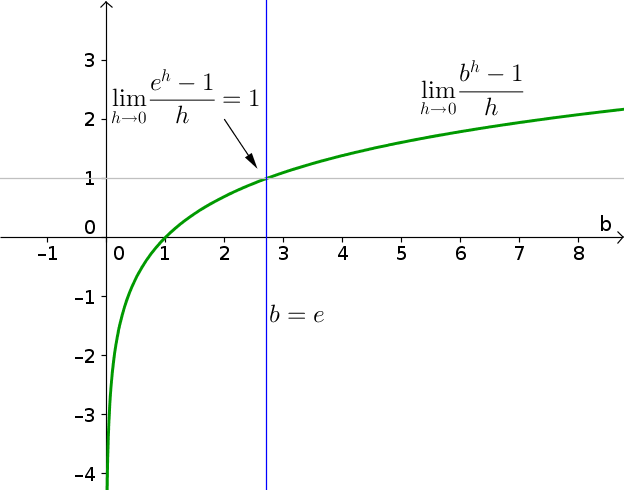# Math Insight

### Image: The limit of e to the h minus 1 over h is equal to oneThe limit $$\lim_{h \to 0} \frac{b^h-1}{h}$$ is equal to one when the base $b$ is equal to the number $e$, as shown by this plot of the limit as a function of the base $b$. The condition that $$\lim_{h \to 0} \frac{e^h-1}{h}=1$$ is one way to define the number $e$.

Image file: limit_e_to_h_minus_1_over_h.png

Source image file: limit_e_to_h_minus_1_over_h.ggb
Source image type: Geogebra